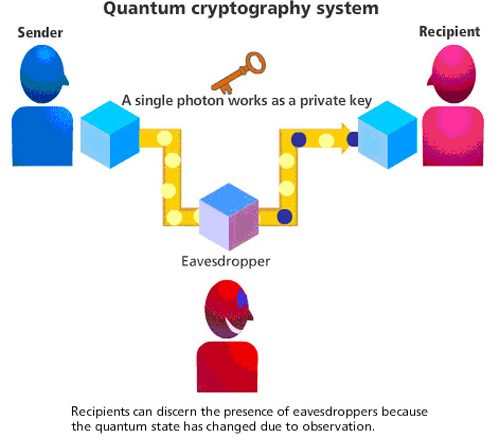# Quantum Cryptography

Quantum Cryptography: In this tutorial, we will briefly study the basis of Quantum cryptography and examples aim to capture. We will cover types of messages in Quantum Cryptography. By Monika Sharma Last updated : May 25, 2023

## What is Quantum Cryptography?

This is a Cryptography which is the study of the science of quantum mechanical properties to perform cryptographic tasks in this way by cryptography. Thus, the main guessing example of quantum cryptography is the quantum key distribution which offers an information-theoretical secure solution to the problem of key exchange in this cryptography to analyze it. The non-quantum communication to do work on it by cryptography. For example, it is impossible to copy data encoded in a quantum state by cryptography. If one attempts to read the encoded data, the quantum state will be changed no-cloning theorem. Thus, this could be used to detect eavesdropping in the quantum key distribution in cryptography.

Whenever Quantum cryptography attributes its beginning by the work of Stephen Wiesner and Gilles Brassard as a publisher. When Wiesner, then at Columbia University in New York, who, in the early 1970s, introduced the concept of quantum conjugate coding in this algorithm. His seminal paper titled "Conjugate Coding" was rejected by the IEEE Information Theory Society, but was eventually published in 1983 in SIGACT News for publishing. Hence, in this paper, he showed how to store or transmit two messages by encoding them in two "conjugate observables", such as linear and circular polarization of photons, so that either, but not both, of which may be received and decoded in this algorithm. Thus, it was not until Charles H.Bennett, of the IBM's Thomas J.Watson Research Center and Gilles Brassard met at the 20th IEEE Symposium held in Puerto Rico that they discovered how to incorporate the findings of Weisner in this way. Basically, in the year 1984 it builds upon this work Bennett and Brassard proposed a method for secure communication, which is now called BB84. Mainly, following a proposal by David Deutsch for using quantum non-locality and Bell's inequalities to achieve secure key distribution in this by Artur Ekert analyzed entanglement-based quantum key distribution in more detail in his 1991 paper for this cryptography.## Working of Quantum Cryptography

Let, assume that two people wish to exchange a message securely, traditionally named Ananya and Bobby as an example. Ananya initiates the message by sending Bobby a key, which will be the mode for encrypting the message data by the cryptography. Mainly, this is a chosen sequence of bits, sent using a certain type of scheme, which can see two different initial values represent one particular binary value as 0 or 1 to make it easy.

Now, let us take that this key is a stream of photons traveling in one direction, with each of these photon particles representing a single bit of data as either a 0 or 1 in this algorithm. Whereas, in addition to their linear travel, all of these photons are oscillating or vibrating in a certain manner to work by it. Thus, these oscillations can occur in any 360-degree range across any conceivable axis, but for the purpose of simplicity as at least as far as it is possible to simplify things in quantum cryptography, let us assume that their oscillations can be grouped into 4 particular states as study: we'll define these as UP/DOWN, LEFT/RIGHT, UPLIFT/RIGHT DOWN and UPRIGHT/LEFT DOWN as the various state. Then, the angle of this vibration is known as the polarization of the photon of quantum. Here, let us introduce a polarizer into the equation by solve cryptography. The same oscillation as before and lets others pass through in a changed state of oscillation as it can also block some photons completely, but let's ignore that property for this exercise like to solve it by cryptography. Ananya has a polarizer that can transmit the photons in any one of the four states mentioned above- in effect, she can choose either rectilinear polarization filters to solve this by cryptography.

Reference: Quantum cryptography